## Legendre's Formula

Counts the number of Positive Integers less than or equal to a numberwhich are not divisible by any of the firstPrimes,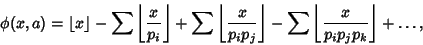(1)

where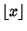is the Floor Function. Taking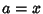gives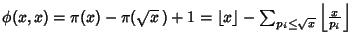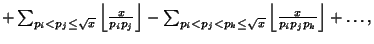(2)
where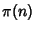is the Prime Counting Function. Legendre's formula holds since one more than the number of Primes in a range equals the number of Integers minus the number of composites in the interval.

Legendre's formula satisfies the Recurrence Relation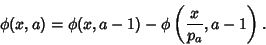(3)

Let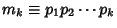, then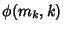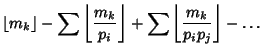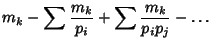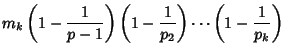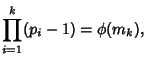(4)

where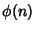is the Totient Function, and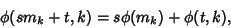(5)

where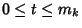. If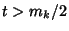, then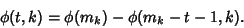(6)

Note that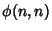is not practical for computingfor large arguments. A more efficient modification is Meissel's Formula.